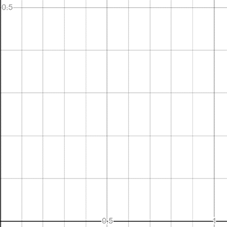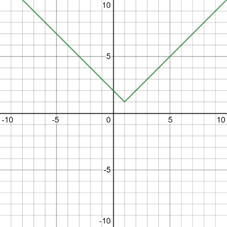# To graph : The equation y = 1 + | x − 1 | is an appropriate viewing rectangle.### Precalculus: Mathematics for Calcu...

6th Edition
Stewart + 5 others
Publisher: Cengage Learning
ISBN: 9780840068071### Precalculus: Mathematics for Calcu...

6th Edition
Stewart + 5 others
Publisher: Cengage Learning
ISBN: 9780840068071

#### Solutions

Chapter 1.9, Problem 21E
To determine

## To graph: The equation y=1+|x−1| is an appropriate viewing rectangle.

Expert Solution

### Explanation of Solution

Given information:

The equation y=1+|x1| .

Graph:

For the graph of equation y=1+|x1| firstly set the suitable values of a,b,c,d for viewing rectangles.

Take values [0,1]×[0,12] then resulting graph is blank because |x1|0 so 1+|x1|1 for all the x .Thus, graph lies above the viewing rectangle, so this viewing rectangle is not appropriate.

The graph of the equation y=1+|x1| can be sketched using the traces,

For thevalue x=0 , the trace of the equation is,

y=1+|01|=1+1=2

For the value x=1 , the trace of the equation is,

y=1+|11|=1

For the plane x=2 , the trace of the equation is,

y=1+|21|=1+1=2

For the different values of x get different values of y .

The whole graph is lies above of the x axes. So, the values of y should be greater or equal to 1.

Take values [-10,10]×[-10,10]The resulting graph is appropriate viewing rectangle.

For enlarge the viewing rectangle change the values of a,b,c,d .

Interpretation:

The graph of an equation in a viewing screen is a viewing rectangle.

The x -values to range from a minimum value of xmin =a to a maximum value of xmax =b

The y -values to range from minimum value of ymin =c to a maximum value of ymax =d

Then, the display portion of the graph lies in the rectangle [a,b]×[c,d]={(x,y)|axb,cyd}

The graph of y=1+|x1| is appropriate in [-10,10]×[-10,10] viewing rectangle

### Have a homework question?

Subscribe to bartleby learn! Ask subject matter experts 30 homework questions each month. Plus, you’ll have access to millions of step-by-step textbook answers!